## Artificial-Intelligence

 Question 1

In Artificial Intelligence (AI), an environment is uncertain if it is

 A Not fully observable and not deterministic B Not fully observable or not deterministic C Fully observable but not deterministic D Not fully observable but deterministic
Artificial-intelligence       Knowledge-representation       UGC-NET CS 2018 JUNE Paper-2
Question 1 Explanation:
→ Deterministic AI environments are those on which the outcome can be determined based on a specific state. In other words, deterministic environments ignore uncertainty.
→ Most real world AI environments are not deterministic. Instead, they can be classified as stochastic. Self-driving vehicles are a classic example of stochastic AI processes.
 Question 2

In Artificial Intelligence (AI), a simple reflex agent selects actions on the basis of

 A current percept, completely ignoring rest of the percept history. B rest of the percept history, completely ignoring current percept. C both current percept and complete percept history. D both current percept and just previous percept.
Artificial-intelligence       Approaches-to-AI       UGC-NET CS 2018 JUNE Paper-2
Question 2 Explanation:
→ Simple reflex agents act only on the basis of the current percept, ignoring the rest of the percept history. The agent function is based on the condition-action rule: "if condition, then action".
→ This agent function only succeeds when the environment is fully observable. Some reflex agents can also contain information on their current state which allows them to disregard conditions whose actuators are already triggered.
→ Infinite loops are often unavoidable for simple reflex agents operating in partially observable environments. Note: If the agent can randomize its actions, it may be possible to escape from infinite loops.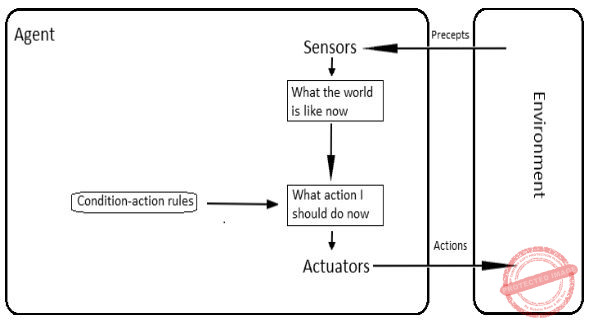Question 3

In heuristic search algorithms in Artificial Intelligence (AI), if a collection of admissible heuristics h1.......hm is available for a problem and none of them dominates any of the others, which should we choose ?

 A h(n) = max{h1 (n), ...., hm(n)} B h(n) = min{h1(n), ...., hm(n)} C h(n) = avg{h1(n), ...., hm(n)} D h(n) = sum{h1(n), ...., hm(n)}
Artificial-intelligence       Planning       UGC-NET CS 2018 JUNE Paper-2
Question 3 Explanation:
Heuristic Search Strategies:
A key component of an evaluation function is a heuristic function h(n), which estimates the cost of the cheapest path from node ‘n’ to a goal node.
→ In connection of a search problem “heuristics” refers to a certain (but loose) upper or lower bound for the cost of the best solution.
→ Goal states are nevertheless identified: in a corresponding node ‘n’ it is required that h(n)=0
E.g., a certain lower bound bringing no information would be to set h(n) ≅ 0
→ Heuristic functions are the most common form in which additional knowledge is imported to the search algorithm.
Generating admissible heuristics from relaxed problems:
→ To come up with heuristic functions one can study relaxed problems from which some restrictions of the original problem have been removed.
→ The cost of an optimal solution to a relaxed problem is an admissible heuristic for the original problem (does not overestimate).
→ The optimal solution in the original problem is, by definition, also a solution in the relaxed problem.
Example:
→ Heuristic h1 for the 8-puzzle gives perfectly accurate path length for a simplified version of the puzzle, where a tile can move anywhere.
→ Similarly h2 gives an optimal solution to a relaxed 8-puzzle, where tiles can move also to occupied squares.
→ If a collection of admissible heuristics is available for a problem, and none of them dominates any of the others, we can use the composite function.
h(n) = max { h1(n), …, hm(n) }
→ The composite function dominates all of its component functions and is consistent if none of the components overestimates. Reference:
http://www.cs.tut.fi/~elomaa/teach/AI-2011-3.pdf
 Question 4

Consider following sentences regarding A*, an informed search strategy in Artificial Intelligence (AI).

(a) A* expands all nodes with f(n)<C*.
(b) A* expands no nodes with f(n)/C*.
(c) Pruning is integral to A*.
Here, C* is the cost of the optimal solution path.
Which of the following is correct with respect to the above statements ?
 A Both statement (a) and statement (b) are true. B Both statement (a) and statement (c) are true. C Both statement (b) and statement (c) are true. D All the statements (a), (b) and (c) are true.
Artificial-intelligence       Genetic-algorithms       UGC-NET CS 2018 JUNE Paper-2
Question 4 Explanation:
A* search:
→ A* combines the value of the heuristic function h(n)and the cost to reach the node ‘n’, g(n).
→ Evaluation function f(n) = g(n) + h(n) thus estimates the cost of the cheapest solution through ‘n’.
→ A* tries the node with the lowest f(n) value first.
→ This leads to both complete and optimal search algorithm, provided that h(n) satisfies certain conditions.
Optimality of A*:
→ A* expands all nodes ‘n’ for which f(n) → However, all nodes n for which f(n) > C* get pruned.
→ It is clear that A* search is complete.
→ A* search is also optimally efficient for any given heuristic function, because any algorithm that does not expand all nodes with f(n) → Despite being complete, optimal, and optimally efficient, A* search also has its weaknesses.
→ The number of nodes for which f(n)< C* for most problems is exponential in the length of the solution.
Reference:
http://www.cs.tut.fi/~elomaa/teach/AI-2011-3.pdf
 Question 5

An agent can improve its performance by

 A Learning B Responding C Observing D Perceiving
Artificial-Intelligence       Knowledge-Representation       UGC-NET CS 2018 DEC Paper-2
Question 5 Explanation:
→ An intelligent agent (IA) is an autonomous entity which observes through sensors and acts upon an environment using actuators (i.e. it is an agent) and directs its activity towards achieving goals (i.e. it is "rational", as defined in economics).
→ Intelligent agents may also learn or use knowledge to achieve their goals. They may be very simple or very complex. A reflex machine, such as a thermostat, is considered an example of an intelligent agent.Question 6
Self Organizing maps are___
 A A type of statistical tool for data analysis B A type of Artificial Swarm networks C A type of particle Swarm algorithm D None of the above
Artificial-intelligence       Self-Organizing-Maps       Nielit Scientist-D 2016 march
Question 6 Explanation:
A self-organizing map or self-organizing feature map is a type of artificial neural network that is trained using unsupervised learning to produce a low-dimensional, discretized representation of the input space of the training samples, called a map, and is therefore a method to do dimensionality reduction. Self-organizing maps differ from other artificial neural networks as they apply competitive learning as opposed to error-correction learning (such as backpropagation with gradient descent), and in the sense that they use a neighborhood function to preserve the topological properties of the input space.
 Question 7
Hopfield networks are a type of__
 A Gigabit network B Terabyte network C Artificial Neural network D Wireless network
Artificial-intelligence       Artificial-neural-networks(ANN)       Nielit Scientist-D 2016 march
Question 7 Explanation:
A Hopfield neural network is a type of artificial neural network invented by John Hopfield in 1982. It usually works by first learning a number of binary patterns and then returning the one that is the most similar to a given input. What defines a Hopfield network: It is composed of only one layer of nodes or units each of which is connected to all the others but not itself. It is therefore a feedback network, which means that its outputs are redirected to its inputs. Every unit also acts as an input and an output of the network. Thus the number of nodes, inputs, outputs of the network are equal. Additionally, each one of the neurons in a has a binary state or activation value, usually represented as 1 or -1, which is its particular output. The state of each node generally converges, meaning that the state of each node becomes fixed after a certain number of updates.
 Question 8
Sigmoidal feedforward artificial neural networks with one hidden layer can / are ___
 A Approximation any continuous function B Approximation any disContinuous function C Approximation any continuous function and its derivatives of arbitrary order. D Exact modeling technique
Artificial-intelligence       Artificial-neural-networks(ANN)       Nielit Scientist-D 2016 march
Question 8 Explanation:
Multilayer perceptron class of networks consists of multiple layers of computational units, usually interconnected in a feed-forward way. Each neuron in one layer has directed connections to the neurons of the subsequent layer. In many applications the units of these networks apply a sigmoid function as an activation function.
A feedforward neural network is an artificial neural network wherein connections between the nodes do not form a cycle. As such, it is different from recurrent neural networks. The feedforward neural network was the first and simplest type of artificial neural network devised. In this network, the information moves in only one direction, forward, from the input nodes, through the hidden nodes (if any) and to the output nodes. There are no cycles or loops in the network.
 Question 9
Match the following :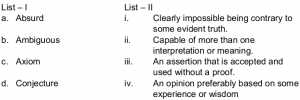A a-i, b-ii, c-iii, d-iv B a-i, b-iii, c-iv, d-ii C a-ii, b-iii, c-iv, d-i D a-ii, b-ii, c-iii, d-iv
Artificial-intelligence       Propositional-Logic       UGC NET CS 2017 Jan -paper-2
Question 9 Explanation:
Absurd→ Clearly impossible being contrary to some evident truth.
Ambiguous→ Capable of more than one interpretation or meaning.
Axiom→ An assertion that is accepted and used without a proof.
Conjecture→ An opinion preferably based on some experience or wisdom
 Question 10
Match the following:A a-i, b-ii, c-iii, d-iv B a-i, b-iii, c-ii, d-iv C a-iii, b-ii, c-iv, d-i D a-ii, b-iii, c-i, d-iv
Artificial-intelligence       Multi-agent-systems       UGC NET CS 2017 Jan -paper-2
Question 10 Explanation:
Affiliate Marketing: Vendors ask partners to place logos on partner’s site. If customers click, come to vendors and buy.
Group Purchasing: Aggregating the demands of small buyers to get a large volume. Then negotiate a price.
Bartering Online: Exchanging surplus products and services with the process administered completely online by an intermediary. Company receives “points” for its contribution.
 Question 11
Let P(m, n) be the statement “m divides n” where the Universe of discourse for both the variables is the set of positive integers. Determine the truth values of the following propositions.
(a)∃m ∀n P(m, n)
(b)∀n P(1, n)
(c) ∀m ∀n P(m, n)
 A (a) - True; (b) - True; (c) - False B (a) - True; (b) - False; (c) - False C (a) - False; (b) - False; (c) - False D (a) - True; (b) - True; (c) - True
Artificial-intelligence       Propositional-Logic       UGC NET CS 2015 Dec- paper-2
Question 11 Explanation:
Given P(m,n) ="m divides n"
Statement-A is ∃m ∀n P(m, n). Here, there exists some positive integer which divides every positive integer. It is true because there is positive integer 1 which divides every positive integer.
Statement-B is ∀n P(1, n). Here, 1 divided every positive integer. It is true.
Statement-C is ∀m ∀n P(m, n). Here, every positive integer divided every positive integer. It is false.
 Question 12
​ Which of the following is true for semi-dynamic ​ environment ?
 A The environment itself does not change with the passage of time but the agent’s performance score does. B The environment change while the agent is deliberating. C Even if the environment changes with the passage of time while deliberating, the performance score does not change. D Environment and performance score, both change simultaneously.
Artificial-intelligence       Semi-Dynamic-Environment       UGC NET CS 2018-DEC Paper-2
Question 12 Explanation:
Semi- Dynamic environment : The environment itself does not change with the passage of time but the agent’s performance score does.
 Question 13
Consider the following statements
S1: ​ A heuristic is admissible if it never overestimates the cost to reach the goal
S2: ​ A heuristic is monotonous if it follows triangle inequality property.
Which one of the following is true referencing the above statements?
 A Statement S1 is true but statement S2 is false. B Statement S1 is false but statement S2 is true. C Neither of the statements S1 and S2 are true D Both the statements S1 and S2 are true.
Artificial-Intelligence       Approches-to-AI       UGC NET CS 2018-DEC Paper-2
Question 13 Explanation:
A heuristic function is said to be admissible if it never overestimates the cost of reaching the goal, i.e. the cost it estimates to reach the goal is not higher than the lowest possible cost from the current point in the path
 Question 14
An agent can improve its performance by
 A Learning B Responding C Observing D Perceiving
Artificial-Intelligence       Knowledge-Representation       UGC NET CS 2018-DEC Paper-2
Question 14 Explanation:
→ An intelligent agent (IA) is an autonomous entity which observes through sensors and acts upon an environment using actuators (i.e. it is an agent) and directs its activity towards achieving goals (i.e. it is "rational", as defined in economics).
→ Intelligent agents may also learn or use knowledge to achieve their goals. They may be very simple or very complex. A reflex machine, such as a thermostat, is considered an example of an intelligent agent.Question 15
In Artificial Intelligence (AI), an environment is uncertain if it is .
 A Not fully observable and not deterministic B Not fully observable or not deterministic C Fully observable but not deterministic D Not fully observable but deterministic
Artificial-Intelligence       Approaches-to-AI       UGC NET CS 2018 JUNE Paper-2
Question 15 Explanation:
→ Deterministic AI environments are those on which the outcome can be determined based on a specific state. In other words, deterministic environments ignore uncertainty.
→ Most real world AI environments are not deterministic. Instead, they can be classified as stochastic. Self-driving vehicles are a classic example of stochastic AI processes.
 Question 16
Back propagation is a learning technique that adjusts weights in the neural network by propagating weight changes.
 A Forward from source to sink B Backward from sink to source C Forward from source to hidden nodes D Backward from since to hidden nodes
Artificial-intelligence       Neural-Networks       UGC NET CS 2012 Dec-Paper-2
Question 16 Explanation:
→ Back propagation is a learning technique that adjusts weights in the neural network by propagating weight changes backward from sink to source.
→ Backpropagation is shorthand for "the backward propagation of errors," since an error is computed at the output and distributed backwards throughout the network’s layers.
 Question 17
​ In Artificial Intelligence (AI), a simple reflex agent selects actions on the basis of .
 A current percept, completely ignoring rest of the percept history. B rest of the percept history, completely ignoring current percept. C both current percept and complete percept history. D both current percept and just previous percept.
Artificial-Intelligence       Knowledge-Representation       UGC NET CS 2018 JUNE Paper-2
Question 17 Explanation:
→ Simple reflex agents act only on the basis of the current percept, ignoring the rest of the percept history. The agent function is based on the ​ condition-action rule ​ : "if condition, then action".
→ This agent function only succeeds when the environment is fully observable. Some reflex agents can also contain information on their current state which allows them to disregard conditions whose actuators are already triggered.
→ Infinite loops are often unavoidable for simple reflex agents operating in partially observable environments. Note: If the agent can randomize its actions, it may be possible to escape from infinite loops.Question 18
In heuristic search algorithms in Artificial Intelligence (AI), if a collection of admissible heuristics h1 .......hm is available for a problem and none of them dominates any of the others, which should we choose ?
 A h(n)=max{h1 (n),....,hm(n)} B h(n)=min{h1 (n),....,hm(n)} C h(n)=avg{h1 (n),....,hm(n)} D h(n)=sum{h1 (n),....,hm(n)}
Artificial-Intelligence       Approaches-to-AI       UGC NET CS 2018 JUNE Paper-2
Question 18 Explanation:
Heuristic Search Strategies:
A key component of an evaluation function is a heuristic function h(n), which estimates the cost of the cheapest path from node ‘n’ to a goal node
→ In connection of a search problem “heuristics” refers to a certain (but loose) upper or lower bound for the cost of the best solution
→ Goal states are nevertheless identified: in a corresponding node ‘n’ it is required that h(n)=0
E.g., a certain lower bound bringing no information would be to set h(n) ≅0
→ Heuristic functions are the most common form in which additional knowledge is imported to the search algorithm Generating admissible heuristics from relaxed problems
→ To come up with heuristic functions one can study relaxed problems from which some restrictions of the original problem have been removed
→ The cost of an optimal solution to a relaxed problem is an admissible heuristic for the original problem (does not overestimate)
→ The optimal solution in the original problem is, by definition, also a solution in the relaxed problem
Example:
→ heuristic h​ 1​ for the 8-puzzle gives perfectly accurate path length for a simplified version of the puzzle, where a tile can move anywhere
→ Similarly h​ 2​ gives an optimal solution to a relaxed 8-puzzle, where tiles can move also to occupied squares
→ If a collection of admissible heuristics is available for a problem, and none of them dominates any of the others, we can use the composite function h(n)=max{ h​ 1​ (n), ..., h​ m​ (n) }
→ The composite function dominates all of its component functions and is consistent if none of the components overestimates.
 Question 19
Consider following sentences regarding A*, an informed search strategy in Artificial Intelligence (AI).
(a) A* expands all nodes with f(n) < C*.
(b) A* expands no nodes with f(n) /C*.
(c) Pruning is integral to A*.
Here, C* is the cost of the optimal solution path.
Which of the following is correct with respect to the above statements ?
 A Both statement (a) and statement (b) are true. B Both statement (a) and statement (c) are true. C Both statement (b) and statement (c) are true. D All the statements (a), (b) and (c) are true.
Artificial-Intelligence       Knowledge-Representation       UGC NET CS 2018 JUNE Paper-2
Question 19 Explanation:
A* search:
→ A* combines the value of the heuristic function h(n)and the cost to reach the node ‘n’, g(n)
→ Evaluation function f(n)=g(n)+h(n) thus estimates the cost of the cheapest solution through ‘n’
→ A* tries the node with the lowest f(n) value first
→ This leads to both complete and optimal search algorithm, provided that h(n) satisfies certain conditions
Optimality of A*
→ A* expands all nodes ‘n’ for which f(n) → However, all nodes n for which f(n) > C* get pruned
→ It is clear that A* search is complete
→ A* search is also optimally efficient for any given heuristic function, because any algorithm that does not expand all nodes with f(n) → Despite being complete, optimal, and optimally efficient, A* search also has its weaknesses.
→ The number of nodes for which f(n)< C* for most problems is exponential in the length of the solution.
 Question 20
Consider a vocabulary with only four propositions A, B, C and D. How many models are there for the following sentence ? B ∨ C
 A 10 B 12 C 15 D 16
Artificial-Intelligence       Knowledge-Representation       UGC NET CS 2018 JUNE Paper-2
Question 20 Explanation:
Here, number of models is nothing but number of TRUEs in final statement. In this proposition logic we got total 15 number of modelsTotal 12 TRUE for given sentence.
 Question 21
Consider the following statements : (a) False╞ True (b) If α╞ (β ∧ γ) then α╞ β and α╞ γ. Which of the following is correct with respect to the above statements ?
 A Both statement (a) and statement (b) are false. B Statement (a) is true but statement (b) is false. C Statement (a) is false but statement (b) is true. D Both statement (a) and statement (b) are true.
Artificial-Intelligence       Knowledge-Representation       UGC NET CS 2018 JUNE Paper-2
Question 21 Explanation:
(a). False╞ True is nothing but False=False|True (or) False=False V True(b). α╞ (β ∧ γ) also write αV(β ∧ γ)
α╞ β and α╞ γ also write (αVβ)∧(αVγ)Finally we proved as LHS=RHS. αV(β ∧ γ) = (αVβ)∧(αVγ)
So, both the statements are correct.
 Question 22
Consider the following two sentences :
(a) The planning graph data structure can be used to give a better heuristic for a planning problem.
(b) Dropping negative effects from every action schema in a planning problem results in a relaxed problem.
Which of the following is correct with respect to the above sentences ?
 A Both sentence (a) and sentence (b) are false. B Both sentence (a) and sentence (b) are true. C Sentence (a) is true but sentence (b) is false D Sentence (a) is false but sentence (b) is true.
Artificial-Intelligence       Approaches-to-AI       UGC NET CS 2018 JUNE Paper-2
Question 22 Explanation:
● Negative effects put restrictions on the action schema. When these restrictions are put into place, then the number of actions that can be taken to get to the next time step decreases because with each addition of a restriction, the actions that do not meet the restriction are filtered out.
● When these negative effects are dropped, then the number of actions increase and dropping all of the negative effects from the action schema results in a relaxed problem.
● A planning graph is a directed graph organized into levels: first a level S​ 0​ for the initial state, consisting of nodes representing each fluent that holds in S​ 0​ ; then a level A​ 0 consisting of nodes for each ground action that might be applicable in S​ 0​ ; then alternating levels S​ i​ followed by A​ i​ ; until we reach a termination condition
● As a tool for generating accurate heuristics, we can view the planning graph as a relaxed problem that is efficiently solvable
 Question 23
A knowledge base contains just one sentence, ∃x AsHighAs (x, Everest). Consider the following two sentences obtained after applying existential instantiation.
(a) AsHighAs (Everest, Everest)
(b) AsHighAs (Kilimanjaro, Everest)
Which of the following is correct with respect to the above sentences ?
 A Both sentence (a) and sentence (b) are sound conclusions. B Both sentence (a) and sentence (b) are unsound conclusions C Sentence (a) is sound but sentence (b) is unsound. D Sentence (a) is unsound but sentence (b) is sound.
Artificial-Intelligence       Knowledge-Representation       UGC NET CS 2018 JUNE Paper-2
Question 23 Explanation:
● The ∃x AsHighAs (x, Everest) means there is one element which as highest as Everest.
● In the statement (a) AsHighAs (Everest, Everest) ,both are Everest then we can’t compare.
● The statement (b) AsHighAs (Kilimanjaro, Everest) means there kilimanjaro which is as highest as Everest So this valid statement.Because we are comparing Kilimanjaro with Everest.
 Question 24
If a process is under statistical control, then it is
 A Maintainable B Measurable C Predictable D Verifiable
Artificial-intelligence       Optimization       UGC NET CS 2012 June-Paper2
Question 24 Explanation:
→ If a process is under statistical control, then it is predictable.
→ Statistical process control(SPC) helps to ensure that the process operates efficiently, producing more specification conforming products with less waste (rework or scrap).
 Question 25
Standard planning algorithms assume environment to be __________.
 A Both deterministic and fully observable B Neither deterministic nor fully observable C Deterministic but not fully observable D Not deterministic but fully observable
Artificial-intelligence       Planning       UGC NET CS 2017 Nov- paper-3
Question 25 Explanation:
→ Classical planning environments that are fully observable, deterministic, finite, static and discrete (in time, action, objects and effects).
 Question 26
Entropy of a discrete random variable with possible values {x1, x2, ..., xn} and probability density function P(X) is :The value of b gives the units of entropy. The unit for b=10 is :
 A bits B bann C nats D deca
Artificial-intelligence       Entropy       UGC NET CS 2017 Nov- paper-3
 Question 27
For any binary (n, h) linear code with minimum distance (2t+1) or greaterA 2t+1 B t+1 C t D t-1
Artificial-intelligence       Linear-Distance       UGC NET CS 2017 Nov- paper-3
 Question 28
Consider a Takagi - Sugeno - Kang (TSK) Model consisting rules of the form: If Xi is Ai1 and... and xr is Air THEN y = fi(x1, x2,.... xr) = bi0 + bi1x1 + birxr assume, ai is the matching degree of rule i, then the total output of the model is given by:
 ABCD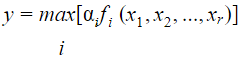Artificial-intelligence       Takagi-Sugeno-Kang (TSK)-Model       UGC NET CS 2017 Nov- paper-3
 Question 29
Consider a single perceptron with sign activation function. The perceptron is represented by weight vector [0.4 −0.3 0.1]t and a bias θ = 0. If the input vector to the perceptron is X = [0.2 0.6 0.5] then the output of the perceptron is:
 A 1 B 0 C -0.25 D -1
Artificial-intelligence       Perceptron       UGC NET CS 2017 Nov- paper-3
 Question 30
Consider the following AO graph:Which is the best node to expand next by AO* algorithm?
 A A B B C C D B and C
Artificial-intelligence       AO* -algorithm       UGC NET CS 2017 Jan- paper-3
Question 30 Explanation:f(n)=c(n)+h(n)
where
f(n)= Best path to reach the destination node
c(n)=Cost of path
h(n)= heuristic value of a node
Cost of choosing B or C is
=(22+3)+(24+2)
=51
Note: We add cost of B and C because they belongs to AND graph.
Cost of choosing A =42+4=46
Since cost of choosing node choosing B or C that’s why we will expand ‘A’.
 Question 31
In Artificial Intelligence (AI), what is present in the planning graph?
 A Sequence of levels B Literals C Variables D Heuristic estimates
Artificial-intelligence       Planning       UGC NET CS 2017 Jan- paper-3
Question 31 Explanation:
In Artificial Intelligence (AI), Sequence of levels is present in the planning graph.
→ A planning graph consists of a sequence of levels that correspond to time steps in the LEVELS plan, where level 0 is the initial state.
→ Each level contains a set of literals and a set of actions.
→ The literals are all those that could be true at that time step, depending on the actions executed at preceding time steps.
→ The actions are all those actions that could have their preconditions satisﬁed at that time step, depending on which of the literals actually hold.
→ The planning graph records only a restricted subset of the possible negative interactions among actions i.e.., it might be optimistic about the minimum number of time steps required for a literal to become true.
→ This number of steps in the planning graph provides a good estimate of how difﬁcult it is to achieve a given literal from the initial state.
→ The planning graph is deﬁned in such a way that it can be constructed very efﬁciently.
→ Planning graphs work only for propositional planning problems-ones with no variables.
 Question 32
What is the best method to go for the game playing problem?
 A Optimal Search B Random Search C Heuristic Search D Stratified Search
Artificial-intelligence       Heuristic-Search       UGC NET CS 2017 Jan- paper-3
Question 32 Explanation:
→ Heuristic search is the best method to go for the game playing problem
→ A heuristic is a method that might not always find the best solution but is guaranteed to find a good solution in reasonable time.
Example: Hill Climbing, Simulated Annealing, Best first search,A* algorithm,etc..,
 Question 33
Let R and S be two fuzzy relations defined as :Then, the resulting relation, T, which relates elements of universe x to the elements of universe z using max-min composition is given by :
 A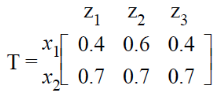B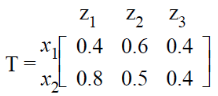CDArtificial-intelligence       Fuzzy-sets       UGC NET CS 2017 Jan- paper-3
Question 33 Explanation:Question 34
A neuron with 3 inputs has the weight vector [0.2 –0.1 0.1]T and a bias θ = 0. If the input vector is X = [0.2 0.4 0.2]T then the total input to the neuron is :
 A 0.20 B 1.0 C 0.02 D –1.0
Artificial-intelligence       Neural-Networks       UGC NET CS 2017 Jan- paper-3
Question 34 Explanation:
Total input to neuron f(x)=(WTXX)+Ⲑ
=(0.2*0.2)+(-0.1*0.4)+(0.1*0.2)
=0.04-0.04+0.02
=0.02
 Question 35
Which of the following neural networks uses supervised learning ?
(A) Multilayer perceptron
(B) Self organizing feature map
(C) Hopfield network

 A (A) only B (B) only C (A) and (B) only D (A) and (C) only
Artificial-intelligence       Neural-Networks       UGC NET CS 2017 Jan- paper-3
Question 35 Explanation:Question 36
Let R and S be two fuzzy relations defined as:Then, the resulting relation, T, which relates elements of universe X to elements of universe Z using max-min composition is given by

 ABCDArtificial-intelligence       Fuzzy-sets       UGC NET CS 2016 July- paper-3
Question 36 Explanation: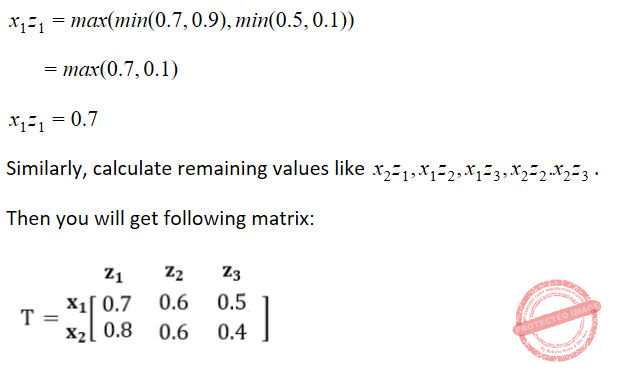Question 37
Compute the value of adding the following two fuzzy integers :
A = {(0.3, 1), (0.6, 2), (1, 3), (0.7, 4), (0.2, 5)}
B = {(0.5, 11), (1, 12), (0.5, 13)}
Where fuzzy addition is defined as μA+B (z)= maxx + y = z(min (μA(x), μB(x)))
Then, f (A + B) is equal to
 A {(0.5, 12), (0.6, 13), (1, 14), (0.7, 15), (0.7, 16), (1, 17), (1, 18)} B {(0.5, 12), (0.6, 13), (1, 14), (1, 15), (1, 16), (1, 17), (1, 18)} C {(0.3, 12), (0.5, 13), (0.5, 14), (1, 15), (0.7, 16), (0.5, 17), (0.2, 18)} D {(0.3, 12), (0.5, 13), (0.6, 14), (1, 15), (0.7, 16), (0.5, 17), (0.2, 18)}
Artificial-intelligence       Fuzzy-sets       UGC NET CS 2016 July- paper-3
Question 37 Explanation:Question 38
A perceptron has input weights W1 = – 3.9 and W2 = 1.1 with threshold value T = 0.3. What output does it give for the input x1 = 1.3 and x2 = 2.2 ?
 A – 2.65 B – 2.30 C 0 D 1
Artificial-intelligence       Neural-Networks       UGC NET CS 2016 July- paper-3
Question 38 Explanation:Question 39
How does randomized hill-climbing choose the next move each time?
 A It generates a random move from the moveset, and accepts this move. B It generates a random move from the whole state space, and accepts this move. C It generates a random move from the moveset, and accepts this move only if this move improves the evaluation function. D It generates a random move from the whole state space, and accepts this move only if this move improves the evaluation function.
Artificial-intelligence       Hill-Climbing-Algorithm       UGC NET CS 2016 July- paper-3
Question 39 Explanation:
Randomized hill-climbing generates a random move from the moveset, and accepts this move only if this move improves the evaluation function.
 Question 40
Consider the following game tree in which root is a maximizing node and children are visited left to right. What nodes will be pruned by the alpha-beta pruning ?A I B HI C CHI D GHI
Artificial-intelligence       Alpha-Beta pruning       UGC NET CS 2016 July- paper-3
 Question 41
Consider a 3-puzzle where, like in the usual 8-puzzle game, a tile can only move to an adjacent empty space. Given the initial statewhich of the following state cannot be reached?
 ABC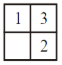DArtificial-intelligence       3-Puzzle-Game       UGC NET CS 2016 July- paper-3
Question 41 Explanation:
This problem, we can solve it by presence of mind.Question 42
A software program that infers and manipulates existing knowledge in order to generate new knowledge is known as
 A Data dictionary B Reference mechanism C Inference engine D Control strategy
Artificial-intelligence       Inference-Engine       UGC NET CS 2016 July- paper-3
Question 42 Explanation:
→ A software program that infers and manipulates existing knowledge in order to generate new knowledge is known as inference engine.

→ Inference engines work primarily in one of two modes either special rule or facts: forward chaining and backward chaining.

→ Forward chaining starts with the known facts and asserts new facts.

→ Backward chaining starts with goals, and works backward to determine what facts must be asserted so that the goals can be achieved

 Question 43
What are the following sequence of steps taken in designing a fuzzy logic machine?
 A Fuzzi cation → Rule evaluation → Defuzzi cation B Fuzzi cation → Defuzzi cation → Rule evaluation C Rule evaluation → Fuzzi cation → Defuzzi cation D Rule evaluation → Defuzzi cation → Fuzzi cation
Artificial-intelligence       Fuzzy-sets       UGC NET CS 2016 Aug- paper-3
Question 43 Explanation:Question 44
Let R and S be two fuzzy relations defined asThen, the resulting relation, T, which relates elements of universe of X to elements of universe of Z using max-product composition is given by
 ABC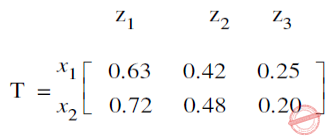DArtificial-intelligence       Fuzzy-sets       UGC NET CS 2016 Aug- paper-3
Question 44 Explanation:Question 45
Match each Artificial Intelligence term in List-I that best describes a given situation in List-IIA I-d, II-a, III-b, IV-c B I-d, II-c, III-a, IV-b C I-d, II-c, III-b, IV-a D I-c, II-d, III-a, IV-b
Artificial-intelligence       Knowledge-representation       UGC NET CS 2016 Aug- paper-3
Question 45 Explanation:
Semantic network→ A method of knowledge representation that uses a graph
Frame→ A data structure representing stereotypical knowledge
Declarative knowledge→ Knowledge about what to do as opposed to how to do it
Primitive→ A premise of a rule that is not concluded by any rule
 Question 46
In Artificial Intelligence , a semantic network
 A is a graph-based method of knowledge representation where nodes represent concepts and arcs represent relations between concepts. B is a graph-based method of knowledge representation where nodes represent relations between concepts and arcs represent concepts. C represents an entity as a set of slots and associated rules. D is a subset of rst-order logic.
Artificial-intelligence       Semantic-Network       UGC NET CS 2016 Aug- paper-3
Question 46 Explanation:
→ In Artificial Intelligence , a semantic network is a graph-based method of knowledge representation where nodes represent concepts and arcs represent relations between concepts.
→ Semantic networks are used in natural language processing applications such as semantic parsing and word-sense disambiguation.
 Question 47
Which formal system provides the semantic foundation for Prolog?
 A Predicate calculus B Lambda calculus C Hoare logic D Propositional logic
Artificial-intelligence       Prolog       UGC NET CS 2016 Aug- paper-3
Question 47 Explanation:
Predicate calculus provides the semantic foundation for Prolog.
 Question 48
Given the following set of prolog clauses :

father(X, Y) :

parent(X, Y),

male(X),

parent(Sally, Bob),

parent(Jim, Bob),

parent(Alice, Jane),

parent(Thomas, Jane),

male(Bob),

male(Jim),

female(Salley),

female(Alice).

How many atoms are matched to the variable ‘X’ before the query father(X, Jane) reports a Result ?

 A 1 B 2 C 3 D 4 E No option is correct.
Artificial-intelligence       Prolog       UGC NET CS 2015 Dec - paper-3
Question 48 Explanation:
Excluded for evaluation
 Question 49
Forward chaining systems are __________ where as backward chaining systems are __________.
 A Data driven, Data driven B Goal driven, Data driven C Data driven, Goal driven D Goal driven, Goal driven
Artificial-intelligence       backward chaining       UGC NET CS 2015 Dec - paper-3
Question 49 Explanation:
Forward Chaining: Forward chaining starts with the available data and uses inference rules to extract more data until a conclusion is reached.
It is also known as data driven inference technique.
It is bottom up reasoning.
It is a breadth first search.
For Example: “If it is raining then i will bring the umbrella”. Here “it is raining” is a available data from which more data is extracted and a conclusion “i will bring the umbrella” is drived.
Backward Chaining: Backward chaining is an inference method described colloquially as working backward from the goal.
It is also known as goal driven inference technique.
Here we starts from a goal and apply inference rules to get some data.
It is top down reasoning.
It is a depth first search.
For Example: “If it is raining then i will bring the umbrella”. Here our conclusion is “i will bring the umbrella”. Now If I am bringing an umbrella then it can be stated that it is raining that is why I am bringing the umbrella. So here “ It is raining” is the data obtained from goal . Hence it was derived in a backward direction so it is the process of backward chaining.
 Question 50
Reasoning strategies used in expert systems include __________.
 A Forward chaining, backward chaining and problem reduction B Forward chaining, backward chaining and boundary mutation C Forward chaining, backward chaining and back propagation D Backward chaining, problem reduction and boundary mutation
Artificial-intelligence       Expert-Systems       UGC NET CS 2015 Dec - paper-3
Question 50 Explanation:
Expert systems: are designed to solve complex problems by reasoning through bodies of knowledge, represented mainly as if–then rules rather than through conventional procedural code.
So we can say that expert systems are used for problem reduction.
For problem reduction an expert system can use forward or backward chaining.
Forward Chaining: Forward chaining starts with the available data and uses inference rules to extract more data until a conclusion is reached.
It is also known as data driven inference technique.
It is bottom up reasoning.
It is a breadth first search.
Backward Chaining: Backward chaining is an inference method described colloquially as working backward from the goal.
It is also known as goal driven inference technique.
Here we starts from a goal and apply inference rules to get some data.
It is top down reasoning.
It is a depth first search.
 Question 51
Language model used in LISP is __________.
 A Functional programming B Logic programming C Object oriented programming D All of the above
Artificial-intelligence       LISP       UGC NET CS 2015 Dec - paper-3
Question 51 Explanation:
LISP is functional language. A functional language:
Uses declarative programming model.
Focus is on “ what you are doing”
Supports parallel programming.
It’s functions have no side effects.
Supports both "Abstraction over Data" and "Abstraction over Behavior".
 Question 52
Consider the two class classification task that consists of the following points:
Class C1 : [-1, -1], [-1, 1], [1, -1]
Class C2 : [1, 1]
The decision boundary between the two classes C1 and C2 using single perceptron is given by:
 A x1 - x2 - 0.5 = 0 B - x1 + x2 - 0.5 = 0 C 0.5(x1 + x2) - 1.5 = 0 D x1 + x2 - 0.5 = 0
Artificial-intelligence       Perceptron       UGC NET CS 2015 Dec - paper-3
Question 52 Explanation:
For such questions,
➜ You should perform multiplication and addition operation between each class matrix and given equations in options.
➜ Option which divides the class into two regions i.e., (+ve & -ve) regions is the correct answer.
Class C1:Question 53
Consider a standard additive model consisting of rules of the form of
If x is Ai AND y is Bi THEN z is Ci.
Given crisp inputs x = x0, y = y0, the output of the model is:
 AB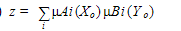C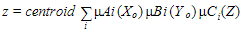DArtificial-intelligence       Additive-Model       UGC NET CS 2015 Dec - paper-3
 Question 54
A bell-shaped membership function is specified by three parameters (a, b, c) as follows:
 A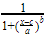BCDArtificial-intelligence       Fuzzy-logic       UGC NET CS 2015 Dec - paper-3
Question 54 Explanation:Question 55
Consider the following:
(a) Evolution
(b) Selection
(c) reproduction
(d) Mutation
Which of the following are found in genetic algorithms?
 A (b),(c) and (d) only B (b) and (d) only C (a),(b),(c) and (d) D (a),(b) and (d) only
Artificial-intelligence       Genetic-algorithms       UGC NET June-2019 CS Paper-2
Question 55 Explanation:
Five phases are considered in a genetic algorithm.
1.Initial population
2.Fitness function
3.Selection
4.Crossover
5.Mutation Note: According to official key option-C is correct.
 Question 56
Which of the following is an example of unsupervised neural network?
 A Back propagation network B Hebb network C Associative memory network D Self-organizing feature map
Artificial-intelligence       Artificial-neural-networks(ANN)       UGC NET June-2019 CS Paper-2
Question 56 Explanation:
A self-organizing map (SOM) or self-organizing feature map (SOFM) is a type of artificial neural network (ANN) that is trained using unsupervised learning to produce a low-dimensional (typically two-dimensional), discretized representation of the input space of the training samples, called a map, and is therefore a method to do dimensionality reduction. Self-organizing maps differ from other artificial neural networks as they apply competitive learning as opposed to error-correction learning (such as backpropagation with gradient descent), and in the sense that they use a neighborhood function to preserve the topological properties of the input space.
 Question 57
The STRIPS representation is
 A a feature-centric representation B an action-centric representation C a combination of feature-centric and action-centric representation D a hierarchical feature-centric representation
Artificial-intelligence       STRIPS       UGC NET June-2019 CS Paper-2
Question 57 Explanation:
The STRIPS representation for an action consists of
→The precondition, which is a set of assignments of values to features that must be true for the action to occur, and
→The effect, which is a set of resulting assignments of values to those primitive features that change as the result of the action.
 Question 58A (a)-(iii), (b)-(iv), (c)-(i), (d)-(ii) B (a)-(iii), (b)-(iv), (c)-(ii), (d)-(i) C (a)-(iv), (b)-(iii), (c)-(i), (d)-(ii) D (a)-(iv), (b)-(iii), (c)-(ii), (d)-(i)
Artificial-intelligence       Knowledge-representation       UGC NET CS 2015 June Paper-3
Question 58 Explanation:
Intelligence → Judgemental
Knowledge → Codifiable, endorsed with relevance and purpose
Information → Scattered facts, easily transferable
Data → Contextual, tacit, transfer needs learning
 Question 59A (a)-(i), (b)-(iv), (c)-(iii), (d)-(ii) B (a)-(iv), (b)-(i), (c)-(ii), (d)-(iii) C (a)-(i), (b)-(iv), (c)-(ii), (d)-(iii) D (a)-(iv), (b)-(ii), (c)-(i), (d)-(iii)
Artificial-intelligence       Hill-Climbing-Algorithm       UGC NET CS 2015 June Paper-3
Question 59 Explanation:
Steepest – accent Hill Climbing→ Considers all moves from current state and selects best move.
Branch – and – bound → Keeps track of all partial paths which can be a candidate for further exploration
Constraint satisfaction → Discover problem state(s) that satisfy a set of constraints
Means – end – analysis → Detects difference between current state and goal state
 Question 60

Let Wo represents weight between node i at layer k and node j at layer (k – 1) of a given multilayer perceptron. The weight updation using gradient descent method is given by

Where α and E represents learning rate and Error in the output respectively?
 ABCD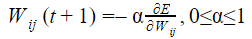Question 61
Consider the following:
(a) Trapping at local maxima
(b) Reaching a plateau
(c) Traversal along the ridge.
Which of the following option represents shortcomings of the hill climbing algorithm?
 A (a) and (b) only B (a) and (c) only C (b) and (c) only D (a), (b) and (c)
Artificial-intelligence       Hill-Climbing-Algorithm       UGC-NET DEC-2019 Part-2
Question 61 Explanation:
Hill climbing limitations:
1. Local Maxima: Hill-climbing algorithm reaching the vicinity a local maximum value, gets drawn towards the peak and gets stuck there, having no other place to go.
2. Ridges: These are sequences of local maxima, making it difficult for the algorithm to navigate.
3. Plateaux: This is a flat state-space region. As there is no uphill to go, algorithm often gets lost in the plateau.
To avoid above problems using 3 standard types of hill climbing algorithm is
1. Stochastic Hill Climbing selects at random from the uphill moves. The probability of selection varies with the steepness of the uphill move.
2. First-Choice Climbing implements the above one by generating successors randomly until a better one is found.
3. Random-restart hill climbing searches from randomly generated initial moves until the goal state is reached.
 Question 62

According to Dempster-Shafer theory for uncertainty management,
Where Bel(A) denotes Belief of event A.
 ABCD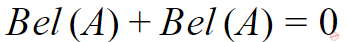Artificial-intelligence       Dempster–Shafer-Theory       UGC-NET DEC-2019 Part-2
 Question 63

Given U={1,2,3,4,5,6,7}

A={(3,0.7),(5,1),(6,0.8)}

then ~A will be: (where ~ → complement)
 A {(4,0.7), (2,1), (1,0.8)} B {(4,0.3), (5,0), (6,0.2)} C {(1,1), (2,1), (3, 0.3), (4, 1), (6, 0.2), (7, 1)} D {(3, 0.3), (6.0.2)}
Artificial-intelligence       Fuzzy-sets       UGC NET CS 2014 June-paper-3
Question 63 Explanation: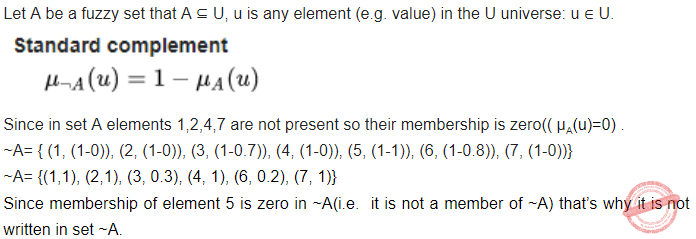Question 64
Consider a fuzzy set old as defined below Old = {(20, 0.1), (30, 0.2), (40, 0.4), (50, 0.6), (60, 0.8), (70, 1), (80, 1)} Then the alpha-cut for alpha = 0.4 for the set old will be
 A {(40, 0.4)} B {50, 60, 70, 80} C {(20, 0.1), (30, 0.2)} D {(20, 0), (30, 0), (40, 1), (50, 1), (60, 1), (70, 1), (80, 1)}
Artificial-intelligence       Fuzzy-sets       UGC NET CS 2014 June-paper-3
Question 64 Explanation:
If the membership of an element is greater than or equal to alpha-cut value then its membership will become one in the resultant set otherwise it’s membership will be zero(i.e. Will not be a member of the resulting set).
Here alpha = 0.4
So new set = {(20, 0), (30, 0), (40, 1), (50, 1), (60, 1), (70, 1), (80, 1)}
OR
{(40, 1), (50,1), (60.1), (70,1), (80,1)}
 Question 65
Perceptron learning, Delta learning and LMS learning are learning methods which falls under the category of
 A Error correction learning – learning with a teacher B Reinforcement learning – learning with a critic C Hebbian learning D Competitive learning – learning without a teacher
Artificial-intelligence       Artificial-neural-networks(ANN)       UGC NET CS 2014 June-paper-3
 Question 66

Which one of the following describes the syntax of prolog program ?

I. Rules and facts are terminated by full stop (.)

II. Rules and facts are terminated by semicolon (;)

 A I, II B III, IV C I, III D II, IV
Artificial-intelligence       Prolog       UGC NET CS 2014 June-paper-3
Question 66 Explanation:
Rules:Question 67

Match the following:

List-I List-II

a. Expert systems i. Pragmatics

b. Planning ii. Resolution

c. Prolog iii. Means-end analysis

d. Natural language processing iv. Explanation facility
 A a-iii, b-iv, c-i, d-ii B a-iii, b-iv, c-ii, d-i C a-i, b-ii, c-iii, d-iv D a-iv, b-iii, c-ii, d-i
Artificial-intelligence       Approaches-to-AI       UGC NET CS 2014 June-paper-3
Question 67 Explanation:
Expert systems → Explanation facility
Planning → Means-end analysis
Prolog → Resolution
Natural language processing → Pragmatics
 Question 68
STRIPS addresses the problem of efficiently representing and implementation of a planner. It is not related to which one of the following ?
 A SHAKEY B SRI C NLP D None of these
Artificial-intelligence       Natural-language-processing       UGC NET CS 2014 June-paper-3
Question 68 Explanation:
STRIPS is related SHAKEY,SRI but not NLP.
 Question 69
Slots and facets are used in
 A Semantic Networks B Frames C Rules D All of these
Artificial-intelligence       Knowledge-representation       UGC NET CS 2014 June-paper-3
Question 69 Explanation:
Slots and facets are used in frames
 Question 70
An A* algorithm is a heuristic search technique which
 A is like a depth-first search where most promising child is selected for expansion B generates all successor nodes and computes an estimate of distance (cost) from start node to a goal node through each of the successors. It then chooses the successor with shortest cost. C saves all path lengths (costs) from start node to all generated nodes and chooses shortest path for further expansion. D none of the above
Artificial-intelligence       AO* -algorithm       UGC NET CS 2014 Dec - paper-3
 Question 71A a-i, b-iii, c-iv, d-ii B a-iv, b-iii, c-i, d-ii C a-i, b-iii, c-ii, d-iv D a-iv, b-i, c-iii, d-ii
Artificial-intelligence       Knowledge-representation       UGC NET CS 2014 Dec - paper-3
 Question 72

Consider the following statements about a perception :

I. Feature detector can be any function of the input parameters
.
II. Learning procedure only adjusts the connection weights to the output layer.

Identify the correct statement out of the following :
 A I is false and II is false. B I is true and II is false. C I is false and II is true. D I is true and II is true.
Artificial-intelligence       Perceptron       UGC NET CS 2014 Dec - paper-3
 Question 73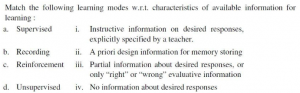A a-i, b-ii, c-iii, d-iv B a-i, b-iii, c-ii, d-iv C a-ii, b-iv, c-iii, d-i D a-ii, b-iii, c-iv, d-i
Artificial-intelligence       Supervised-And-Unsupervised-Learning       UGC NET CS 2014 Dec - paper-3
 Question 74
Consider the statement below. A person who is radical (R) is electable (E) if he/she is conservative (C), but otherwise is not electable. Few probable logical assertions of the above sentence are given below.Which of the above logical assertions are true? Choose the correct answer from the options given below:
 A (B) only B (C) only C (A) and (C) only D (B) and (D) only
Artificial-intelligence       Propositional-Logic       UGC NET JRF November 2020 Paper-2
Question 74 Explanation:
1. (R ∧E) ↔C
This is not equivalent. It says that all (and only) conservatives are radical and electable.
2. R →(E ↔C)
This one is equivalent. if a person is a radical then they are electable if and only if they are conservative.
3. R →((C →E) ∨¬E)
This one is vacuous. It’s equivalent to ¬R ∨ (¬C ∨ E ∨ ¬E), which is true in all interpretations.
4.R ⇒ (E ⇐⇒ C) ≡ R ⇒ ((E ⇒ C) ∧ (C ⇒ E))
≡ ¬R ∨ ((¬E ∨ C) ∧ (¬C ∨ E))
≡ (¬R ∨ ¬E ∨ C) ∧ (¬R ∨ ¬C ∨ E))
There are 74 questions to complete.